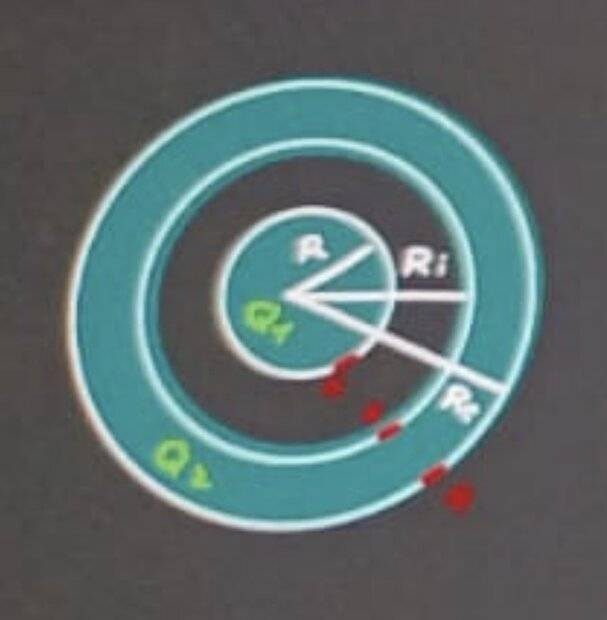# Potential difference metallic sphere

• Guillem_dlc
In summary, the conversation discusses the calculation of electric potential difference using the TOTAL influence theorem and the convention of defining the zero level as the potential at infinity. The formula used for the potential at the surface of a uniformly charged sphere is also mentioned. The conversation also includes a humorous anecdote about Van de Graaff and his interest in rockets.

#### Guillem_dlc

Homework Statement
A metallic sphere of radius ##R=3,0\, \textrm{cm}## is located concentrically inside a spherical crust also metallic of inner radius ##R_i=10\, \textrm{cm}## and outer radius ##R_e=12\, \textrm{cm}##. The sphere has a charge ##Q_1=+1,2\, \textrm{nC}## and the clean charge of the crust is ##Q_2=+6\, \textrm{nC}##. Calculate:
a) The charge on the inner and outer surfaces of the crust.
b) Sphere potential and crustal potential (consider ##V_\infty=0##)
The bark is then connected to the ground. Calculate:
c) The charge of the sphere and that of the outer and inner surfaces of the crust.
d) Sphere potential and crustal potencial.

Sol: a) ##Q_{2i}=-1,2\, \textrm{nC}##; ##Q_{2e}=+7,2\, \textrm{nC}##
b) ##V_{\textrm{crustal}} = 540\, \textrm{V}##; ##V_{\textrm{sphere}}=790\, \textrm{V}##
c) ##Q_1=+1,2\, \textrm{nC}##; ##Q_{2i}=-1,2\, \textrm{nC}##; ##Q_{2e}=0##
d) ##V_{\textrm{crustal}}=0##; ##V_{\textrm{sphere}}=250\, \textrm{V}##
Relevant Equations
Total influence theorem (a)a) We know that ##Q_1=1,2\, \textrm{nC}## and ##Q_2=6\, \textrm{nC}##. By the TOTAL influence theorem:
$$-Q_1=Q_{2i}=-1,2\, \textrm{nC}$$
$$Q_2=Q_{2i}+Q_{2e}\rightarrow Q_{2e}=7,2\, \textrm{nC}$$

b) Electric potential difference crust:
$$V_A-V_\infty=$$

•Delta2
Guillem_dlc said:
Not quite sure what you are asking. What formula would you normally use for the potential at the surface of a uniformly charged sphere, charge Q radius r?
Potentials are relative. What convention does the formula assume wrt the zero potential?

haruspex said:
Not quite sure what you are asking. What formula would you normally use for the potential at the surface of a uniformly charged sphere, charge Q radius r?
Potentials are relative. What convention does the formula assume wrt the zero potential?
There, I remember how I did it. What do you mean by convention?

Guillem_dlc said:
There, I remember how I did it. What do you mean by convention?
Please quote the formula you are using.

ref: https://en.wikipedia.org/wiki/Van_de_Graaff_generator

Just anecdotal, and hopefully humorous ##-## my grandfather was a litte older than Van de Graaff, and they were friends ##-## VdG (5 years old at the time) was interested in rockets, and one day he saw a car accelerating rapidly down the street, and he observed the exhaust pipe, and he asked Grandpa "how does that little pipe push that car so fast", and Grandpa explained to him that/how it wasn't the thrust from the exhaust pipe that gave the impetus to the car ##\dots##

Last edited:
haruspex said:
Please quote the formula you are using.
b) Crust:
$$V_A-V_\infty=-\int_\infty^A E\, dl=-\int_\infty^A E\, dr=$$
$$=-\int_\infty^A kQ_1+Q_2\dfrac{1}{r^2}\, dr=+\left( \dfrac{k(Q_1+Q_2)}{R_e}\right)=540\, \textrm{V}$$

Sphere: ##V_C-V_\infty =(V_C-V_B)+\overbrace{(V_A-V_\infty)}^{=540}##
$$V_C-V_B=-\int_B^C E\, dr=-\int_B^C \dfrac{kQ_1}{r^2}\, dr=-\left[ kQ_1\left( \dfrac{1}{R_1}-\dfrac{1}{R_i}\right) \right]=$$
$$=250\, \textrm{V} \rightarrow V_C-V_\infty =790\, \textrm{V}$$

Guillem_dlc said:
b) Crust:
$$V_A-V_\infty=-\int_\infty^A E\, dl=-\int_\infty^A E\, dr=$$
$$=-\int_\infty^A kQ_1+Q_2\dfrac{1}{r^2}\, dr=+\left( \dfrac{k(Q_1+Q_2)}{R_e}\right)=540\, \textrm{V}$$

Sphere: ##V_C-V_\infty =(V_C-V_B)+\overbrace{(V_A-V_\infty)}^{=540}##
$$V_C-V_B=-\int_B^C E\, dr=-\int_B^C \dfrac{kQ_1}{r^2}\, dr=-\left[ kQ_1\left( \dfrac{1}{R_1}-\dfrac{1}{R_i}\right) \right]=$$
$$=250\, \textrm{V} \rightarrow V_C-V_\infty =790\, \textrm{V}$$
Ok, so in each case what you have calculated is a difference from ##V_\infty##.
Strictly speaking, voltage is always a matter of potential difference. There is no absolute potential.
A common convention is to define the zero level as the potential at infinity. That is not always applicable, e.g. in analysing infinite charged plates, but it is fine where all the charges are in a bounded region. You are instructed to use that convention here. Hence ##V_\infty=0##.

•Guillem_dlc
haruspex said:
Ok, so in each case what you have calculated is a difference from ##V_\infty##.
Strictly speaking, voltage is always a matter of potential difference. There is no absolute potential.
A common convention is to define the zero level as the potential at infinity. That is not always applicable, e.g. in analysing infinite charged plates, but it is fine where all the charges are in a bounded region. You are instructed to use that convention here. Hence ##V_\infty=0##.